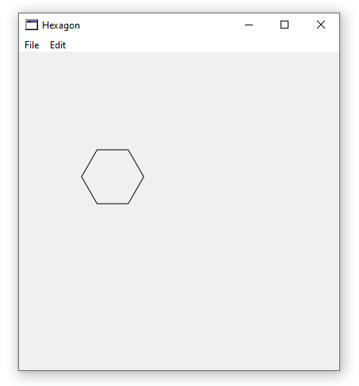# C# to Xojo

I tried to convert the C code in http://csharphelper.com/blog/2015/10/draw-a-hexagonal-grid-in-c/ to Xojo
The original code should create an array of points to draw an hexagon.
I’m probably doing something wrong because it does not work.
Any suggestion welcome.

//=====

[code]Function HexToPoints(height As double, row As Double, col As double) As double()
dim width as double = HexWidth(height)
dim y as double = height/2
dim x as double = 0

// Move down the required number of rows.
y = y + (row * height)

// If the column is odd, move down half a hex more.
if (col mod 2) = 1 then
y = y + (height /2)
end if

// Move over for the column number.
x = x + (col * (width * 0.75))

dim pointF() as double
pointF.Append x
pointF.Append y

pointF.Append (x + width) * 0.25
pointF.Append (y - height) / 2

pointF.Append (x + width) * 0.75
pointF.Append (y - height) / 2

pointF.Append (x + width)
pointF.Append y

pointF.Append (x + width) * 0.75
pointF.Append (y + height) / 2

pointF.Append (x + width) * 0.25
pointF.Append (y + height) / 2

return PointF
End Function[/code]

//=========

```Function HexWidth(height as double) As double return (4 * (height/2/Sqrt(3))) End Function```

The most beloved error “it doesn’t work”. What doesn’t work? How do you use the grid? You got an example project?

Well, the point array created do not paint an hexagon. (I do not know how to post an image here)

[code]Sub Paint(g As Graphics, areas() As REALbasic.Rect) Handles Paint
Dim points() As Double

points = HexToPoints(68,1,1)
g.DrawPolygon(points)

End Sub
[/code]

then copy the bblink and paste it in your message (between img tags)Sorry, I do not saw an image, so I was thinking at an error and I added its link above. The error is local: my ISP filter imgur links from this forum ! (not the direct one !).As your code is drawing, but not correctly, you must have the point calculations wrong.
If you take this line for example:

``new PointF(x + width * 0.25f, y - height / 2)``

you translated the latter half to

``pointF.Append (y - height) / 2``

By putting `y - height` in brackets, you change the calculation order which in the original code is `y - (height/2)`.
Look for these places in your code and make sure you are doing the original calculations.

Hi Enric,

There were two issues with the example, 1) HexToPoints had the brackets in the wrong position, 2) differences between a 0-based array and a 1-based array.

```pointF.Append (x + width) * 0.25 (incorrect) pointF.Append (x + width * 0.25) (correct)```

DrawPolygon starts drawing an array at point 1, not point 0. I added the following line of code in HexToPoints to just add a value in the zero-position:

``pointF.Append 0 //1-based array``